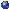Events

Junior Applied Math And ProbabilityLindsay Martin, RLM 9.166: Equivalent extensions of Hamilton-Jacobi-Bellman equations defined on hypersurfaces into Euclidean space
Friday, March 08, 2019, 02:00pm - 03:00pm
I will present a new formulation for the computation of solutionsof a class of Hamilton Jacobi Bellman (HJB) equations on closedsmooth surfaces of co-dimension one. It is well known that theviscosity solution of the HJB equation equivalent to the valuefunction of a corresponding optimal control control problem.In this talk, I will show how we extend the optimal control problemgiven on the surface to an equivalent one defined in a sufficientlysmall narrow band of that co-dimensional one surface in the ambientEuclidean space. The extension is done appropriately so thatthe corresponding HJB equation, in the narrow band, has viscositysolutions which are identical to the constant normal extensionof the value functions of the original optimal control problem.Then we can use existing numerical methods developed on Cartesiangrids to solve HJB equations on surfaces. Some numerical resultswill also be presented.Location: RLM 9.166